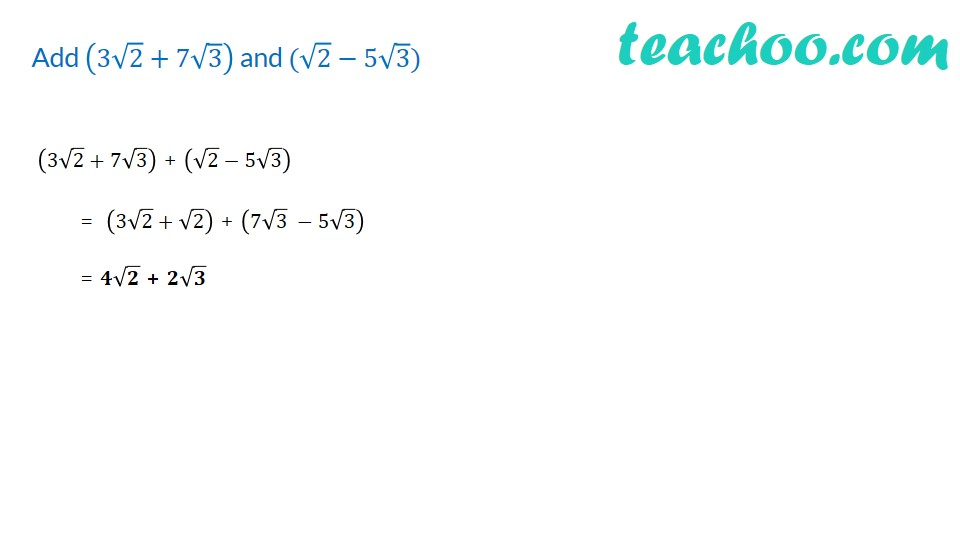1. Chapter 1 Class 9 Number Systems (Term 1)
2. Concept wise
3. Rationalising

Transcript

Add (3√2+7√3) and (√2−5√3) (3√2+7√3) + (√2−5√3) = (3√2+√2) + (7√3 −5√3) = 𝟒√𝟐 + 𝟐√𝟑

Rationalising